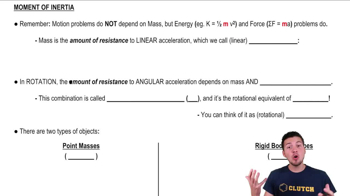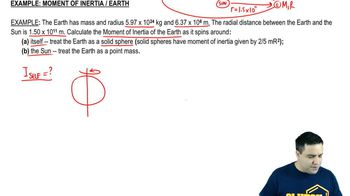Start typing, then use the up and down arrows to select an option from the list.
5:29 minutes
Problem 9a
Textbook Question

# CALC A slender rod with length L has a mass per unit length that varies with distance from the left end, where x = 0, according to dm/dx = gx, where g has units of kg/m^2. (b) Use Eq. (9.20) to calculate the moment of inertia of the rod for an axis at the left end, perpendicular to the rod. Use the expression you derived in part (a) to express I in terms of M and L. How does your result compare to that for a uniform rod? Explain.Verified Solution
This video solution was recommended by our tutors as helpful for the problem above.
91views